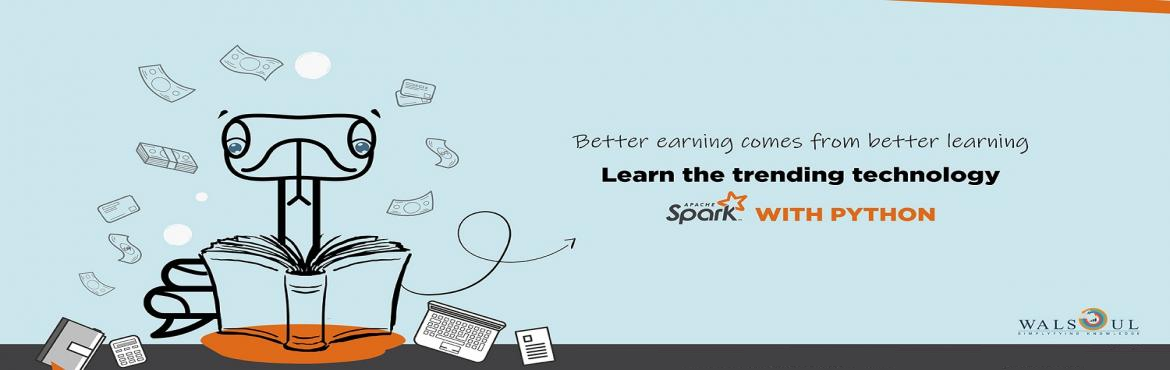# Spark with Python - Weekend Course - 6 Hours a Day - 24 Hours Course

• ## Online and Offline

Sale Date Ended

INR 12000
Sold Out

#### Invite friends

Page Views : 8

Overview

Apache Spark with Python training will advance your expertise in Distributed programming with Spark and Python. Skill set gained through the course in Core Spark, Python, SparkSQL and Streaming will help you to solve complex problem. Deep knowledge of Spark with Python will always make you distinct, which will open a successful path for your career.

Objective

Hadoop mapreduce faciliteted to solve complex problems on distributed systems but with some limitations. This course will discuss limitation of Hadoop mapreduce and how Spark overcomes those limitations. We describe RDDs which is core of Spark and In memory computation. Understanding of persistent RDDs, in memory computation, and solving Big Data problems using Spark with Python is core of this course. Discussion will move through SparkSQL and problem solving with SparkSQL dataframes. Hand-on is the parallel movement for all the discussion. Concept on dealing with streaming data with Spark  Streaming is also an important topic, which is included. Last part of course is Spark program optimization. Optimization of Spark core, Spark SQL,  Spark streaming and optimizing the utilization of cluster system . We discuss Spark on Yarn, Standalone and Mesos cluster too. Training will go through many small projects to get you working on Spark clusters.

Day wise distribution of class :

1. Day 1 : Python, Bigdata , Spark Introduction and Component of Spark ,Operation on Single RDD,
2. Day 2 : Operation on Paired RDD, Fault tolerance and Persistence, Optimizing spark code, IO in spark
3. Day 3 : Spark Streaming, SparkSQL

Detail Deion of class :

Introduction to Big Data and Distributed Computing :

Big data analysis is future. This section of course will help you to understand, the need of distributed computation.

Introduction to data.

Data Science a vision.

• Introduction to Data.
• Big data Introduction.
• Big Data use cases.
• DFS and problem.
• Parallel computation.
• Problem with parallel computation.

• HDFS and its architecture.
• HDFS Commands
• mkdir
• ls
• rmdir and rm
• copyFromLocal
• put
• cat
• copyToLocal
• get
• touchz
• mv
• cp
• distcp
• etc…...
• fsimage and edits log files.
• Introduction to MapReduce.
• Shortcoming of MapReduce.

Python : Refresher

• Introduction to Python.
• Jupytor
• Python variables and Data Type.
• Operators in Python.
• Interactive mode and base programming introduction
• Python Collections (List, Dictionaries etc)
• Control Flow and looping in Python
• Functions in Python (Declaration, Definition Types and calling)
• Object oriented Python.
• NumPy

Spark Introduction :

• Introduction to Spark.
• Spark and Hadoop (Similarity and Differences).
• Spark Execution (Master Slave System , Drive, Driver manager and Executors).
• Spark Shell.
• Resilient Distributed dataSet (RDD).

Operations On RDD :

• Creation of RDD
• Transformation and Action Introduction
• Lazy evaluation
• Some Important Transformation :
• Filter
• map
• flatMap
• distinct
• sample
• union
• intersection
• subtract
• cartesian
• Some Important Action
• first
• take
• top
• reduce
• fold
• foreach
• count
• collect

Paired RDD :

• Introduction and usefulness of Paired RDD.
• Some important Transformation on pairRDD.
• combineBy
• mapValues
• groupByKeys
• reduceByKeys
• sortByKeys
• subsractByKey
• Joines and their Type
• cogroup
• Some Important action on pair RDD
• lookUp
• collectAsMap
• countByKey
• Hands on all the functions.

Fault tolerance and Persistence :

• RDD lineage
• persistence
• Benefit of persistence

Optimizing Spark program :

• Introduction to partitioning
• Inbuilt partitioners (Hash and Range)
• Benefits of partitioning
• groupByKey and reduceBykey comparison

IO in Spark :

• TextFile
• Csv File
• JSON
• Data From HDFS

Spark Streaming :

• Introduction to Streaming Data.
• Component of Spark Streaming.
• Transformation
• Window Concept
• Kafka integration with Streaming.
• State management.
• Performance

SparkSQL.

• Introduction to SparkSQL
• SparkSQL datatype
• DataFrame an Introduction.
• Creation of a dataframe.
• Summary statistics on DataFrame.
• Aggregation on Given Data.
• SparkSQL and SQL
• Introduction to Hive.
• Using data from Hive and HiveQL.
• Optimizing SparkSQL code.

Spark Code Deployment and cluster managers :

• Submitting Spark code on StandAlone cluster manager.
• Submitting Spark code on YARN
• Submitting Spark code on Mesos

Note : Every part of course will be associated with hands on . A number of objective questions will always help you in scratch your brain.

Projects :

Project 1 : Spark core can be used for data preparation and aggregation. Aggregation will be implemented using Spark core APIs.
For data aggregation movie lance data will be used.

Project 2 : Implementing streaming data word frequency visualization. using Kafka and Spark streaming integration.

Project 3 : Implementation of Moving average using SparkSQL.

Project 4 : Data preprocessing, data manipulation and aggregation using SparkSQL. It will be done using Real time data.

Note:  This course is both online as well as offline, offline classroom claasess will be conducted at BTM Bangalore